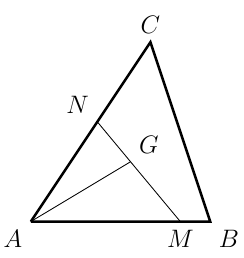AM/GM VectorMethod 希望杯 Intermediate
2011

Problem - 2959

Let $G$ be the centroid of $\triangle{ABC}$. Points $M$ and $N$ are on side $AB$ and $AC$, respectively such that $\overline{AM} = m\cdot\overline{AB}$ and $\overline{AN} = n\cdot\overline{AC}$ where $m$ and $n$ are two positive real numbers. Find the minimal value of $mn$.The solution for this problem is available for $0.99. You can also purchase a pass for all available solutions for$99.